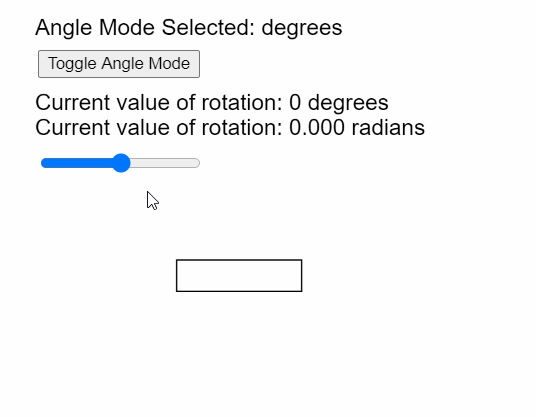Open in App
Not now

# p5.js | angleMode() Function

• Last Updated : 31 May, 2020

The angleMode() function in p5.js is used to set the mode in which the angle is interpreted. It can be set to either degrees or radians. All the functions that use an angle as a parameter would follow the angle mode set by this function.

Syntax:

```angleMode( mode )
```

Parameters: This function accept a single parameter as mentioned above and described below:

• mode: It is a constant that can be used to set the mode in which the angle would be interpreted. It can have the values of DEGREES or RADIANS.

The example below illustrates the angleMode() function in p5.js:
Example:

## javascript

 `let angleModeSelected; ` ` `  `function` `setup() { ` `  ``createCanvas(400, 300); ` `  ``textSize(18); ` ` `  `  ``// Set the default angle to DEGREES ` `  ``angleModeSelected = DEGREES; ` ` `  `  ``// Create a button for toggling the angle mode ` `  ``angleModeToggler = createButton(``"Toggle Angle Mode"``); ` `  ``angleModeToggler.position(30, 40); ` `  ``angleModeToggler.mouseClicked(() => { ` `    ``if` `(angleModeSelected == DEGREES) angleModeSelected = RADIANS; ` `    ``else` `angleModeSelected = DEGREES; ` `  ``}); ` ` `  `  ``// Create a slider for changing the current angle ` `  ``angleSlider = createSlider(-180, 180, 0, 1); ` `  ``angleSlider.position(30, 120); ` `} ` ` `  `function` `draw() { ` `  ``clear(); ` ` `  `  ``// Get the angle from the slider ` `  ``let angleToRotate = angleSlider.value(); ` ` `  `  ``// Convert the angle to radians ` `  ``// for demonstration ` `  ``let angleInRadians = (angleToRotate / 57.295).toFixed(3); ` ` `  `  ``text(``"Angle Mode Selected: "` `+ angleModeSelected, 20, 20); ` `  ``text(``"Current value of rotation: "` `+ angleToRotate + ``" degrees"``, 20, 80); ` `  ``text(``"Current value of rotation: "` `+ angleInRadians + ``" radians"``, 20, 100); ` ` `  `  ``// Set the angle mode ` `  ``// based on the selected mode ` `  ``angleMode(angleModeSelected); ` ` `  `  ``translate(width / 3, height / 1.5); ` ` `  `  ``// Rotate the shape according to ` `  ``// the angle specified ` `  ``rotate(angleToRotate); ` ` `  `  ``// Draw the rectangle that ` `  ``// would be rotated ` `  ``rect(0, 0, 100, 25); ` `} `

Output:Online editor: https://editor.p5js.org/
Environment Setup: https://www.geeksforgeeks.org/p5-js-soundfile-object-installation-and-methods/
Reference: https://p5js.org/reference/#/p5/angleMode

My Personal Notes arrow_drop_up
Related Articles